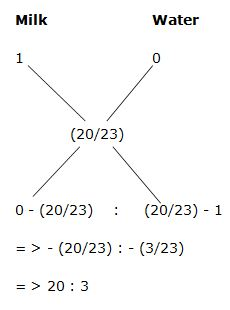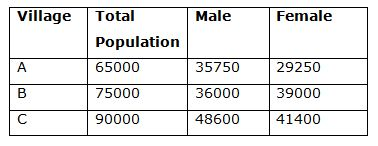# LIC AAO/SBI PO Prelims Quantitative Aptitude Questions 2019 (Day-23)

Dear Aspirants, Our IBPS Guide team is providing new series of Quantitative Aptitude Questions for LIC AAO/SBI PO 2019 so the aspirants can practice it on a daily basis. These questions are framed by our skilled experts after understanding your needs thoroughly. Aspirants can practice these new series questions daily to familiarize with the exact exam pattern and make your preparation effective.

[WpProQuiz 5910]

### Click Here for SBI PO Pre 2019 High-Quality Mocks Exactly on SBI Standard

1) A and B can separately complete a piece of work in 24 days and 27 days respectively. After how many days should B leave so that the work gets completed in 16 days, if both start working simultaneously?

a) 12 days

b) 15 days

c) 10 days

d) 9 days

e) None of these

2) A and B entered into a partnership by investing Rs. 28000 and Rs. 35000 respectively. After 4 months, A withdraws two-seventh of the initial investment but B invested Rs. 5000 more. And after another 3 months, C entered into a partnership with Rs. 50000. Find the share of B, if the total profit at the end of the year is Rs. 171850?

a) Rs. 80500

b) Rs. 72800

c) Rs. 85600

d) Rs. 76400

e) None of these

3) If the radius of the circle is seven-ninth of side of the square. The perimeter of the square is 72 meter. Find the area of the circle?

a) 784 Sq m

b) 652 Sq m

c) 616 Sq m

d) 736 Sq m

e) None of these

4) In what ratio, water will be mixed with milk to earn a profit of 15 % by selling the mixture at cost price?

a) 15 : 4

b) 17 : 5

c) 18 : 7

d) 20 : 3

e) None of these

5) 3 years ago, the ratio of ages of Raj and Kamal is 5 : 6. The age of Jothi after 7 years is 45 years. 3 years ago, the age of Raj is five-seventh of age of Jothi at that time. Find the present age of Kamal?

a) 28 years

b) 33 years

c) 36 years

d) 24 years

e) None of these

Directions (Q. 6 – 10) Study the following information carefully and answer the given questions:

There are 3 villages (A, B and C) in a certain city. The total population of those 3 villages are 2.3 lakhs. The population of village C is 20 % more than the population of village B and the population of village A is 65000. The percentage of male population in village A and B is 55 % and 48 % respectively. Total female population in village C is 48600 less than the total population in that village.

6) Find the difference between the total literate population in village A to that of total female population in village B, if ratio between the literate to illiterate population in village A is 7 : 6?

a) 4000

b) 7500

c) 9000

d) 6000

e) None of these

7) Find the ratio between the total male population in village A to that of total illiterate population in village B, if the percentage of literate population in village B is 75 %?

a) 225 : 77

b) 52 : 19

c) 65 : 14

d) 143 : 75

e) None of these

8) Find the average number of female population in all the given villages together?

a) 34800

b) 36550

c) 35400

d) 37250

e) None of these

9) Total population in village C is what percentage of total male population in village B?

a) 250 %

b) 175 %

c) 230 %

d) 190 %

e) None of these

10) Find the difference between the total male population to that of female population in all the given villages together?

a) 12500

b) 11600

c) 10700

d) 13200

e) None of these

According to the question,

(16/24) + (x/27) = 1

(2/3) + (x/27) = 1

(X/27) = 1 – (2/3)

(X/27) = 1/3

X = 9 days

The share of A, B and C,

= > [28000*4 + 28000*(5/7)*8] : [35000*4 + 40000*8] : [50000*5]

= > [112000 + 160000] : [140000 + 320000] : 

= > 272000 : 460000 : 250000

= > 136 : 230 : 125

491’s = 171850

1’s = 350

The share of B = 230’s = Rs. 80500

The perimeter of the square = 72 m

4a = 72

Side (a) = 18 m

Radius of the circle = (7/9)*18 = 14 m

Area of the circle = πr2 = (22/7)*14*14 = 616 Sq m

Let the cost price be Rs. 1

Selling price of mixture = Rs. 1

Cost price of mixture = 1 * 100/115 = 20/233 years ago, the ratio of ages of Raj and Kamal = 5 : 6 (5x, 6x)

The age of Jothi after 7 years = 45 years

3 years ago, the age of Jothi = 35 years

3 years ago, the age of Raj = (5/7)*Jothi = (5/7)*35 = 25

According to the question,

5’s = 25

1’s = 5

The age of Kamal, 3 years ago = 6x = 30

The present age of Kamal = 33 years

Directions (Q. 6 – 10):

The total population of 3 villages = 2.3 lakhs = 230000

The population of village C = (120/100)*the population of village B

Village C : Village B = 6 : 5

The population of village A = 65000

The total population of village B and C together

= > 230000 – 65000 = 165000

The population of village B

= > 165000*(5/11) = 75000

The population of village C

= > 165000*(6/11) = 90000

The total male population in village A

= > 65000*(55/100) = 35750

Total female population in village A = 65000 – 35750 = 29250

The total of male population in village B

= > 75000*(48/100) = 36000

Total female population in village B = 75000 – 36000 = 39000

Total male population in village C = 48600

Total female population in village C = 90000 – 48600 = 41400The total literate population in village A

= > 65000*(7/13) = 35000

The total female population in village B = 39000

Required difference = 39000 – 35000 = 4000

The total male population in village A = 35750

The total illiterate population in village B

= > 75000*(25/100) = 18750

Required ratio = 35750 : 18750 = 143 : 75

The average number of female population in all the given villages together

= > (29250 + 39000 + 41400)/3

= > 109650/3 = 36550

Total population in village C = 90000

Total male population in village B = 36000

Required % = (90000/36000)*100 = 250 %

The total male population in all the given villages together

= > 35750 + 36000 + 48600 = 120350

The total female population in all the given villages together

= > 29250 + 39000 + 41400 = 109650

Required difference = 120350 – 109650 = 10700